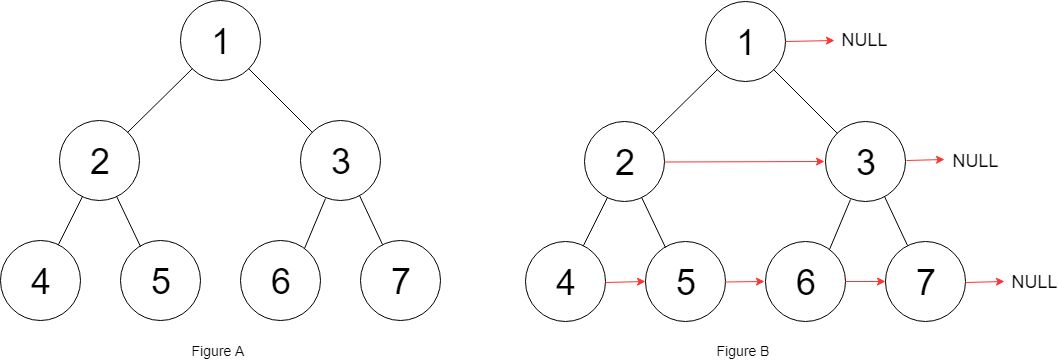# 116. Populating Next Right Pointers in Each Node

You are given a perfect binary tree where all leaves are on the same level, and every parent has two children. The binary tree has the following definition:

``````struct Node {
int val;
Node *left;
Node *right;
Node *next;
}
``````

Populate each next pointer to point to its next right node. If there is no next right node, the next pointer should be set to `NULL`.

Initially, all next pointers are set to `NULL`.

Example:``````Input: {"\$id":"1","left":{"\$id":"2","left":{"\$id":"3","left":null,"next":null,"right":null,"val":4},"next":null,"right":{"\$id":"4","left":null,"next":null,"right":null,"val":5},"val":2},"next":null,"right":{"\$id":"5","left":{"\$id":"6","left":null,"next":null,"right":null,"val":6},"next":null,"right":{"\$id":"7","left":null,"next":null,"right":null,"val":7},"val":3},"val":1}

Output: {"\$id":"1","left":{"\$id":"2","left":{"\$id":"3","left":null,"next":{"\$id":"4","left":null,"next":{"\$id":"5","left":null,"next":{"\$id":"6","left":null,"next":null,"right":null,"val":7},"right":null,"val":6},"right":null,"val":5},"right":null,"val":4},"next":{"\$id":"7","left":{"\$ref":"5"},"next":null,"right":{"\$ref":"6"},"val":3},"right":{"\$ref":"4"},"val":2},"next":null,"right":{"\$ref":"7"},"val":1}

Explanation: Given the above perfect binary tree (Figure A), your function should populate each next pointer to point to its next right node, just like in Figure B.
``````

Note:

• You may only use constant extra space.
• Recursive approach is fine, implicit stack space does not count as extra space for this problem.

``````class Solution {
public:
Node* connect(Node* root) {
if (!root) return NULL;
if (root->left) root->left->next = root->right;
if (root->right) root->right->next = root->next? root->next->left : NULL;
connect(root->left);
connect(root->right);
return root;
}
};
``````

``````// Non-recursion, more than constant space
class Solution {
public:
Node* connect(Node* root) {
if (!root) return NULL;
queue<Node*> q;
q.push(root);
while (!q.empty()) {
int size = q.size();
for (int i = 0; i < size; ++i) {
Node *t = q.front(); q.pop();
if (i < size - 1) {
t->next = q.front();
}
if (t->left) q.push(t->left);
if (t->right) q.push(t->right);
}
}
return root;
}
};
``````

``````// Non-recursion, constant space
class Solution {
public:
Node* connect(Node* root) {
if (!root) return NULL;
Node *start = root, *cur = NULL;
while (start->left) {
cur = start;
while (cur) {
cur->left->next = cur->right;
if (cur->next) cur->right->next = cur->next->left;
cur = cur->next;
}
start = start->left;
}
return root;
}
};
``````

Github 同步地址：

https://github.com/grandyang/leetcode/issues/116

Populating Next Right Pointers in Each Node II

Binary Tree Right Side View

https://leetcode.com/problems/populating-next-right-pointers-in-each-node/

https://leetcode.com/problems/populating-next-right-pointers-in-each-node/discuss/37473/My-recursive-solution(Java)

https://leetcode.com/problems/populating-next-right-pointers-in-each-node/discuss/37472/A-simple-accepted-solution

LeetCode All in One 题目讲解汇总(持续更新中…)

 微信打赏Venmo 打赏×

Help us with donation